【亿码酷站-编程开发教程】收集全网优质教程及源码资源！``` \$sql = "select * from user_info where user_id='".\$_GET['userId']."'";
\$result = mysql_query(\$sql,\$con);
if(\$row = mysql_fetch_array(\$result)){
}```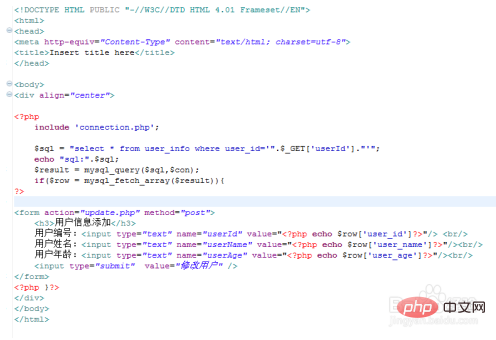```//通过post获取页面提交数据信息
\$userId = \$_POST[userId];
\$userAge = \$_POST[userAge];

\$sql = "update user_info set user_name='".\$userName."',user_age=".\$userAge." where user_id='".\$userId."'";
mysql_query(\$sql,\$conn);//执行SQL
\$mark  = mysql_affected_rows();//返回影响行数
\$url = "userinf_select.php";```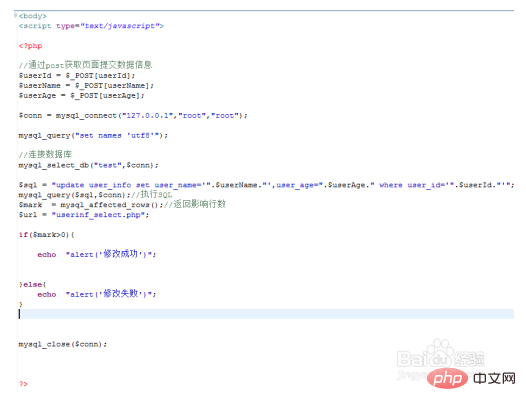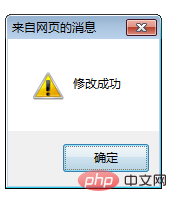```\$userId = \$_GET['userId'];

include 'connection.php';

\$sql = "delete from user_info where user_id='".\$userId."'";

mysql_query(\$sql,\$con);

\$mark  = mysql_affected_rows();//返回影响行数

if(\$mark>0){
echo "删除成功";
}else{
echo  "删除失败";
}

mysql_close(\$con);```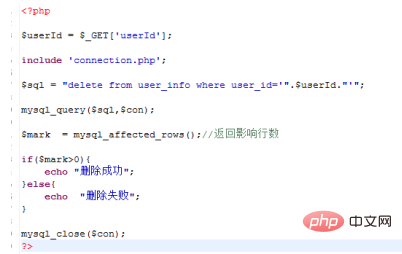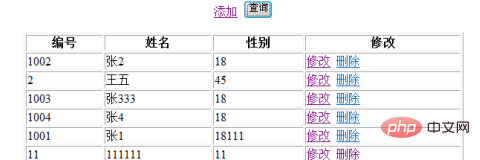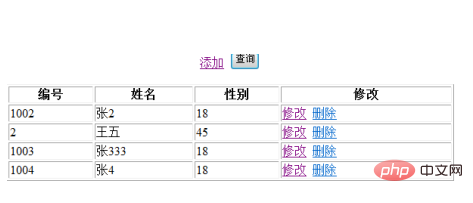php如何修改数据库中数据